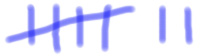THE education site for computer science and ICT

1. Introduction to Numbers

Computers understand the world through binary numbers - sequences of 1s and 0s.

But what is a binary number? How does it relate to the numbers we use every day?

The answer is that both binary and our 'normal' numbers, called denary, are just different representations of the same thing.

What is the number shown below?We are pretty sure that you would have instantly known that it was 7. But how did you know that? It doesn't look anything like a 7.

The point is that a number can be represented as any set of symbols as long as the rules are consistent. What we are basically trying to point out is that a 'number' can be represented in many different forms.

This section will look at how to convert numbers from the form we're most familiar with - denary - into binary and then back again.